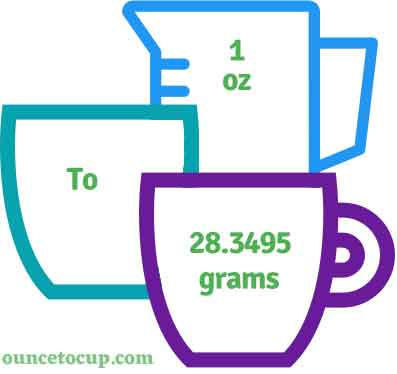# Ounce to Gram (oz to g conversion)

Are you cooking your favorite dish? The detailed chart in the recipe includes the calculation of 1 ounces to g conversion? Do not worry; check this conversion tool to find how many 1 ounces equal to g in a minute. This 1 oz to g converter gives an exact measurement for any recipe you prepare.

Ounce Value:

oz

Gram Value:

g

1 Ounce = 28.3495 Gram
(1 oz = 28.3495 g)

Try our auto 1 ounce to gram calculator (Without Convert Button), Just change the first field value and you got final value.## How many g is a oz?

We know that the volume value of 1 oz is equal to 28.3495 g. If you want to convert 1 fluid oz to an equal number of g, just multiply the volume value by 28.3495. Hence, 1 Ounce is equal to 28.3495 g.

The Answer is: 1 US Fluid Ounces = 28.3495 US g

1 oz = 28.3495 g

Many of them try to search or find an answer for what is 1 ounces in g? So, we’ll start with 1 fl oz to g conversion to know how big is 1 oz.

## How To Calculate 1 fluid oz to g?

To calculate 1 fluid ounces to an equal number of gram, simply follow the steps below.

Fluid Ounces to Grams formula is:

Gram = Fluid Ounce * 28.3495

Assume that we are finding out how many g were found in 1 fl oz of water, multiply by 28.3495 to get the result.

Applying to Formula: g = 1 oz * 28.3495 = 28.3495 g.

## How To Convert oz to g?

• To convert fluid ounces to g,
• Simply multiply the fluid ounce value by 28.3495.
• Applying to the formula, g = 1 ounces * 28.3495 [1x28.3495].
• Hence, 1 ounces is equal to 28.3495 g.

## Some quick table references for ounce to gram conversions:

Ounce [oz]Gram [g]
1 oz28.3495 g
2 oz56.699 g
3 oz85.0485 g
4 oz113.398 g
5 oz141.7475 g
6 oz170.097 g
7 oz198.4465 g
8 oz226.796 g
9 oz255.1455 g
10 oz283.495 g
11 oz311.8445 g
12 oz340.194 g
13 oz368.5435 g
14 oz396.893 g
15 oz425.2425 g

## Reverse Calculation: How many ounces are in a g?

• To convert 1 g to oz,
• Simply divide the 1 g by 28.3495.
• Then, applying the formula, ounce = 1 g / 28.3495 [1/28.3495 = 0.035274].
• Hence, 1 g is equal to 0.035274 oz.

### Related Converter:

Formula: Ounce to Grams

g = ounce * 28.3495

Applying to Formula,

g = 1*28.3495 = 28.3495

1 oz = 28.3495 g